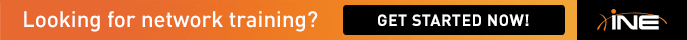# MQC Dynamic Flows and WRED

How the 25 percetage  was configured  to a value of 4 ?

• The last value is the max-probability-denominator

As per the Cisco Document:

http://www.cisco.com/c/en/us/td/docs/ios/qos/command/reference/qos_book/qos_q1.html#wp1054345

Denominator for the fraction of packets dropped when the average queue
depth is at the maximum threshold. For example, if the denominator is
512, 1 out of every 512 packets is dropped when the average queue is at
the maximum threshold. The value range is from 1 to 65536. The default
is 10; 1 out of every 10 packets is dropped at the maximum threshold.

Basically, the answer is 4 because 1 out of every 4 packets will be dropped. (25%)

• How the 25 percetage  was configured  to a value of 4 ?

1/4 =  0.25 = 25% where 4 is the value set for the denominator